# Math in Focus Grade 6 Chapter 1 Lesson 1.1 Answer Key The Number Line

Go through the Math in Focus Grade 6 Workbook Answer Key Chapter 1 Lesson 1.1 The Number Line to finish your assignments.

## Math in Focus Grade 6 Course 1 A Chapter 1 Lesson 1.1 Answer Key The Number Line

### Math in Focus Grade 6 Chapter 1 Lesson 1.1 Guided Practice Answer Key

Draw a horizontal number line to represent each set of whole numbers.

Question 1.
Positive whole numbers less than 5
0, 1, 2, 3 and 4 are the positive whole numbers less than 5.

Question 2.
Whole numbers greater than 9 but less than 14
10, 11, 12, 13 are the whole numbers greater than 9 but less than 14.

Draw a vertical number line to represent each set of whole numbers.

Question 3.
Odd numbers between 1 and 10
1, 3, 5, 7 and 9 are the odd numbers between 1 and 10.

Question 4.
Positive odd numbers < 15
1, 3, 5, 7, 9, 11, 13  are the positive odd numbers < 15.

Represent fractions, mixed numbers, and decimals on a horizontal number line.

a) Represent the fractions $$\frac{1}{4}$$, $$\frac{2}{4}$$, and $$\frac{3}{4}$$ on a number line.To represent $$\frac{1}{4}$$s, subdivide each interval between two consecutive whole numbers into four equal intervals using three tick marks.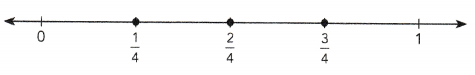b) Represent the decimals from 0.1 to 0.9 on a horizontal number line. Use an interval of 0.1 between each decimal.To represent decimals in tenths, subdivide each interval between two consecutive whole numbers into ten equal intervals.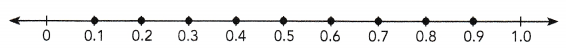Complete each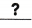with the correct value, and each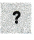with > or <.

Question 5.
Fill in the missing fractions and mixed numbers on the number line. Then complete the statements of inequality.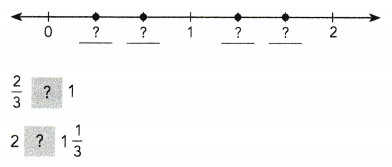Draw a horizontal number line to represent each set of numbers.

Question 6.
Mixed numbers greater than 10 but less than 11 Use an interval of $$\frac{1}{4}$$ between each pair of mixed numbers.

Question 7.
Mixed numbers from 3 to 4, with an interval of $$\frac{1}{10}$$ between each pair of mixed numbers.

Question 8.
Decimals between 0 and 1, with an interval of 0.25 between each pair of decimals

Question 9.
Decimals greater than 8.0 but less than 12.0
Use an interval of 0.8 between each pair of decimals.

Represent fractions1 mixed numbers, and decimals on a vertical number line.

a) Represent the fractions $$\frac{1}{5}$$, $$\frac{2}{5}$$, $$\frac{3}{5}$$, $$\frac{4}{5}$$ on a vertical number line.
To represent these fractions on the number line, subdivide the interval between 0 and 1 into five smaller intervals.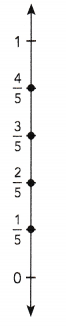b) Represent the decimals 2.0, 2.2, 2.4, 2.6, …, 4.0 on a vertical number line.
To represent these decimals, subdivide the interval from 2.0 to 4.0 into ten equal intervals of 0.2.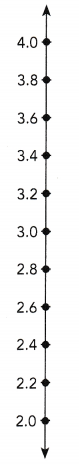To decide how many tick marks to show on the number line, you can 3.6 count up by 0.2s, marking them as you go:
2.0, 2.2, 2.4, 2.6, 2.8, 3.0, 3.2, 3.4, 3.2, 3.6, 3.8, 4.0.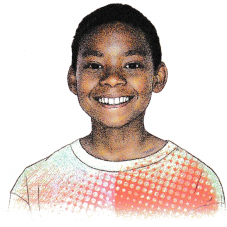Write statements of inequality comparing two fractions or two decimals using the symbols > and <.

You can use a number line to compare fractions and decimals.

a) Compare the two fractions, $$\frac{2}{3}$$ and $$\frac{5}{6}$$.
Use a number line to compare $$\frac{2}{3}$$ and $$\frac{5}{6}$$.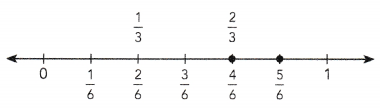The numbers $$\frac{2}{3}$$ and $$\frac{4}{6}$$ are equivalent and are represented by the same point on a number line.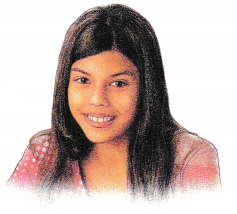The fraction $$\frac{5}{6}$$ lies to the right of $$\frac{2}{3}$$. This means that $$\frac{5}{6}$$ is greater than $$\frac{2}{3}$$.
This can be represented as:
$$\frac{5}{6}$$ > $$\frac{2}{3}$$.

b) Compare the two decimals, 1.3 and 1.15. Use a number line to help you.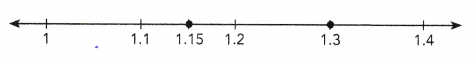The decimal 1.15 lies to the left of 1.3. This means that 1.15 is less than 1.3. This can be represented by:
1.15 < 1.3.

Complete each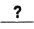with the correct value, and each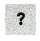with > or <.

Question 10.
a) Fill in the missing decimals on the number line.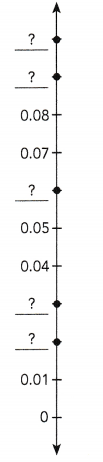b) Compare each pair of decimals using < or >. Use the number line in a) to help you.
0.1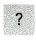0.05 0.020.07

Draw a vertical number line to represent each set of numbers.

Question 11.
Mixed numbers greater than 6 but less than 7
Use an interval of $$\frac{1}{6}$$ between each pair of mixed numbers.

Question 12.
Positive fractions less than 1, with an interval of $$\frac{1}{12}$$ between each pair of fractions.

Question 13.
Decimals between 11.4 and 15.0, with an interval of 0.4 between each pair of decimals

Question 14.
Decimals greater than 7.2 but less than 9.6
Use an interval of 0.3 between each pair of decimals.

Question 15.
Positive decimals less than 7.5, with an interval of 0.75 between each pair of decimals

Compare each pair of numbers using > or <. Use a number line to help you.

Question 16.
3$$\frac{9}{10}$$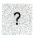3$$\frac{3}{10}$$

Question 17.
2.172.71

Question 18.
14.413.38

Question 19.
8$$\frac{5}{12}$$$$\frac{100}{12}$$

Compare numbers in different forms.

Look at these number lines.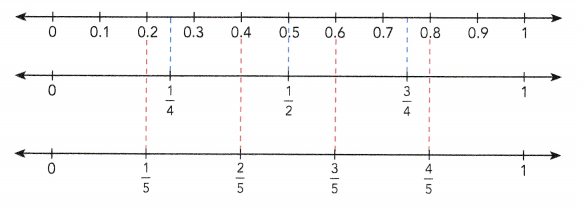Every whole number, fraction, and decimal can be represented on the number line. A given point on a number line can be written ¡n different forms. For example,
$$\frac{1}{2}$$ = 0.5 and $$\frac{3}{4}$$ = 0.75.You can see that $$\frac{1}{4}$$ = 0.25, $$\frac{1}{2}$$ = 0.5, and $$\frac{3}{4}$$ = 0.75.
You can also see that $$\frac{1}{5}$$ = 0.2, $$\frac{2}{5}$$ = 0.4, $$\frac{3}{5}$$ = 0.6, and $$\frac{4}{5}$$ = 0.8.

a) Which is greater, $$\frac{1}{4}$$ or 0.3?
$$\frac{1}{4}$$ = 0.25
0.25 lies to the left of 0.3
So, 0.3 > $$\frac{1}{4}$$

b) Which is lesser, 0.62 or $$\frac{3}{5}$$?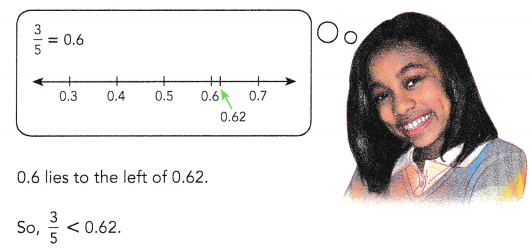Complete.

Question 20.
Which is greater, $$\frac{1}{2}$$ or 0.55?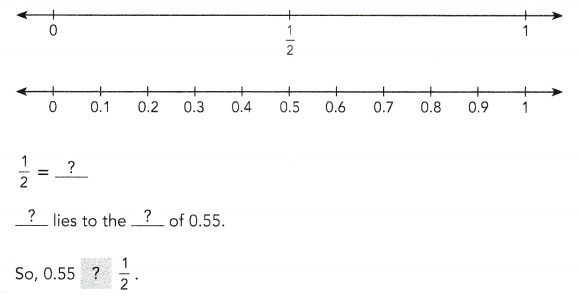Compare each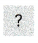using > or <. Use a number line to help you.

Question 21.
0.2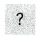$$\frac{1}{4}$$

Question 22.
$$\frac{3}{4}$$0.7

Question 23.
0.89$$\frac{4}{5}$$

Question 24.
0.25$$\frac{1}{5}$$

Question 25.
$$\frac{2}{5}$$0.3

Question 26.
3.263$$\frac{5}{8}$$

### Math in Focus Course 1A Practice 1.1 Answer Key

Copy and complete each number line by filling in the missing values.

Question 1.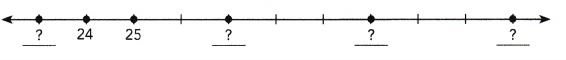Question 2.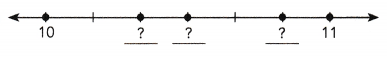Question 3.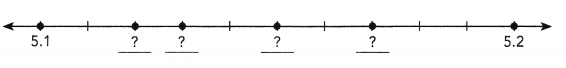Draw a horizontal number line to represent each set of numbers.

Question 4.
Odd numbers from 11 to 21

Question 5.
Positive whole numbers less than 9

Question 6.
Whole numbers greater than 12 but less than 18

Question 7.
Mixed numbers between 0 and 2, with an interval of $$\frac{1}{3}$$ between each pair of mixed numbers

Question 8.
Decimals from 7.0 to 8.4, with an interval of 0.2 between each pair of decimals

Draw a vertical number line to represent each set of numbers.

Question 9.
Even numbers between 20 and 32

Question 10.
Positive whole numbers less than 13

Question 11.
Whole numbers greater than 6 but less than 16

Question 12.
Positive fractions less than 1, with an interval of $$\frac{1}{8}$$ between each pair of fractions

Question 13.
Decimals between 10 and 15, with an interval of 0.75 between each pair of decimals

Compare each pair of numbers using > or <. Use a number line to help you.

Question 14.
2$$\frac{3}{7}$$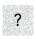1$$\frac{9}{7}$$

Question 15.
$$\frac{17}{25}$$1$$\frac{7}{25}$$

Question 16.
1$$\frac{4}{5}$$1$$\frac{2}{3}$$

Question 17.
33.6136.13

Question 18.
59.0559.5

Question 19.
98.07298.027

Draw a horizontal number line from 2 to 3 to represent each set of numbers.

Question 20.
2$$\frac{4}{5}$$, 2$$\frac{3}{20}$$, 2$$\frac{1}{2}$$, 2$$\frac{11}{20}$$, and 2$$\frac{9}{20}$$

Question 21.
2.5, 2.125, 2.375, and 2.875

Draw a horizontal number line from 0 to 1 to represent each set of numbers.

Question 22.
$$\frac{1}{3}$$, $$\frac{1}{4}$$, $$\frac{3}{8}$$, $$\frac{3}{4}$$, $$\frac{7}{8}$$ and $$\frac{5}{6}$$

Question 23.
0.1, 0.25, 0.05, 0.8, 0.75, and 0.95

Compare each pair of numbers using > or <. Use a number line to help you.

Question 24.
0.8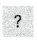$$\frac{1}{10}$$

Question 25.
$$\frac{1}{5}$$0.25

Question 26.
$$\frac{3}{5}$$0.35

Question 27.
0.14$$\frac{1}{4}$$

Question 28.
$$\frac{2}{5}$$0.3

Question 29.
0.64$$\frac{9}{10}$$

Question 30.
0.2$$\frac{1}{6}$$

Question 31.
$$\frac{7}{8}$$0.87
The wingspan of one butterfly is 1$$\frac{9}{16}$$ inches. The wingspan of another butterfly is 1$$\frac{5}{8}$$ inches. Write an inequality comparing the two wingspans.
For a class project, Jina made a model of the Empire State Building that was 23.7 centimeters tall. Her friend Caleb made a model that was 23$$\frac{3}{5}$$ centimeters tall. Whose model was taller? How much taller was it?